﻿ TGD改正对BDS-3新频点单点定位的影响分析文章快速检索 高级检索
 大地测量与地球动力学2023, Vol. 43Issue (5): 447-451  DOI: 10.14075/j.jgg.2023.05.002### 引用本文DU Yanjun, JIA Xiaolin, YAO Wanqiang, et al. Influence of Timing Group Delay on BDS-3 New Signals Single Point Positioning[J]. Journal of Geodesy and Geodynamics, 2023, 43(5): 447-451.### Foundation support

National Natural Science Foundation of China, No.41874041.

### Corresponding author

JIA Xiaolin, researcher, PhD supervisor, majors in satellite navigation, E-mail: 13891907401@139.com.

### 第一作者简介

DU Yanjun, PhD candidate, majors in LEO satellite orbit determination, E-mail: duyanjun@stu.xust.edu.cn.

### 文章历史

TGD改正对BDS-3新频点单点定位的影响分析

1. 西安科技大学测绘科学与技术学院，西安市雁塔中路58号，710054;
2. 西安测绘研究所，西安市雁塔中路1号，710054;
3. 长安大学地质工程与测绘学院，西安市雁塔路126号，710054

1 观测方程及TGD改正公式 1.1 伪距观测方程

 $\begin{gathered} P_{\mathrm{r}}^{\mathrm{s}}=\rho_{\mathrm{r}}^{\mathrm{s}}+c \cdot \mathrm{d} t_{\mathrm{r}}-c \cdot \mathrm{d} t^{\mathrm{s}}+ \\ T_{\mathrm{r}}^{\mathrm{s}}+I_{\mathrm{r}}^{\mathrm{s}}+b_{\mathrm{r}}-b^{\mathrm{s}}+\varepsilon_{\mathrm{r}}^{\mathrm{s}} \end{gathered}$ (1)

 $\begin{gathered} P_{\mathrm{r}, \mathrm{IF}}^{\mathrm{s}}=\alpha \cdot P_{\mathrm{r}, i}^{\mathrm{s}}+\beta \cdot P_{\mathrm{r}, j}^{\mathrm{s}}=\rho_{\mathrm{r}}^{\mathrm{s}}+ \\ c \cdot \mathrm{d} t_{\mathrm{r}}-c \cdot \mathrm{d} t^{\mathrm{s}}+T_{\mathrm{r}}^{\mathrm{s}}+b_{\mathrm{r}, \mathrm{IF}}-b_{\mathrm{IF}}^{\mathrm{s}}+\varepsilon_{\mathrm{r}, \mathrm{IF}}^{\mathrm{s}} \end{gathered}$ (2)

1.2 基于广播星历的TGD改正公式

 $\left\{\begin{array}{l} b_{\mathrm{B} 1 \mathrm{I}}^{\mathrm{s}}=T_{\mathrm{GD} 1} \\ b_{\mathrm{B} 1 \mathrm{C}}^{\mathrm{s}}=T_{\mathrm{GDB} 1 \mathrm{Cp}} \\ b_{\mathrm{B} 2 \mathrm{a}}^{\mathrm{s}}=T_{\mathrm{GDB} 2 \mathrm{ap}} \\ b_{\mathrm{B} 31}^{\mathrm{s}}=0 \end{array}\right.$ (3)

 $\left\{\begin{array}{l} b_{\mathrm{B} 11 / \mathrm{B} 3 \mathrm{I}}^{\mathrm{s}}=\frac{f_{\mathrm{B} 1 \mathrm{I}}^2}{f_{\mathrm{B} 1 \mathrm{I}}^2-f_{\mathrm{B} 3 \mathrm{I}}^2} T_{\mathrm{GD} 1} \\ b_{\mathrm{B} 1 \mathrm{C} / \mathrm{B} 2 \mathrm{a}}^{\mathrm{s}}=\frac{f_{\mathrm{B} 1 \mathrm{C}}^2 T_{\mathrm{GDB} 1 \mathrm{Cp}}-f_{\mathrm{B} 2 \mathrm{a}}^2 T_{\mathrm{GDB} 2 \mathrm{ap}}}{f_{\mathrm{B} 1 C}^2-f_{\mathrm{B} 2 \mathrm{a}}} \\ b_{\mathrm{B} 1 / \mathrm{B} 2 \mathrm{a}}^{\mathrm{s}}=\frac{f_{\mathrm{B} 1 \mathrm{I}}^2 T_{\mathrm{GD1}}-f_{\mathrm{B} 2 \mathrm{a}}^2 T_{\mathrm{GDB} 2 \mathrm{ap}}}{f_{\mathrm{B} 1 \mathrm{I}}^2-f_{\mathrm{B} 2 \mathrm{a}}^2} \\ b_{\mathrm{B} 1 \mathrm{C} / \mathrm{B} 3 \mathrm{I}}^{\mathrm{s}}=\frac{f_{\mathrm{B} 1 \mathrm{C}}^2}{f_{\mathrm{B} 1 \mathrm{C}}^2-f_{\mathrm{B} 3 \mathrm{I}}^2} T_{\mathrm{GDB} 1 \mathrm{Cp}} \end{array}\right.$ (4)
2 实验与分析 2.1 数据来源及处理策略

2.2 DCB稳定性及与TGD的符合度图 1 CAS DCB产品时间序列 Fig. 1 Time series of CAS DCB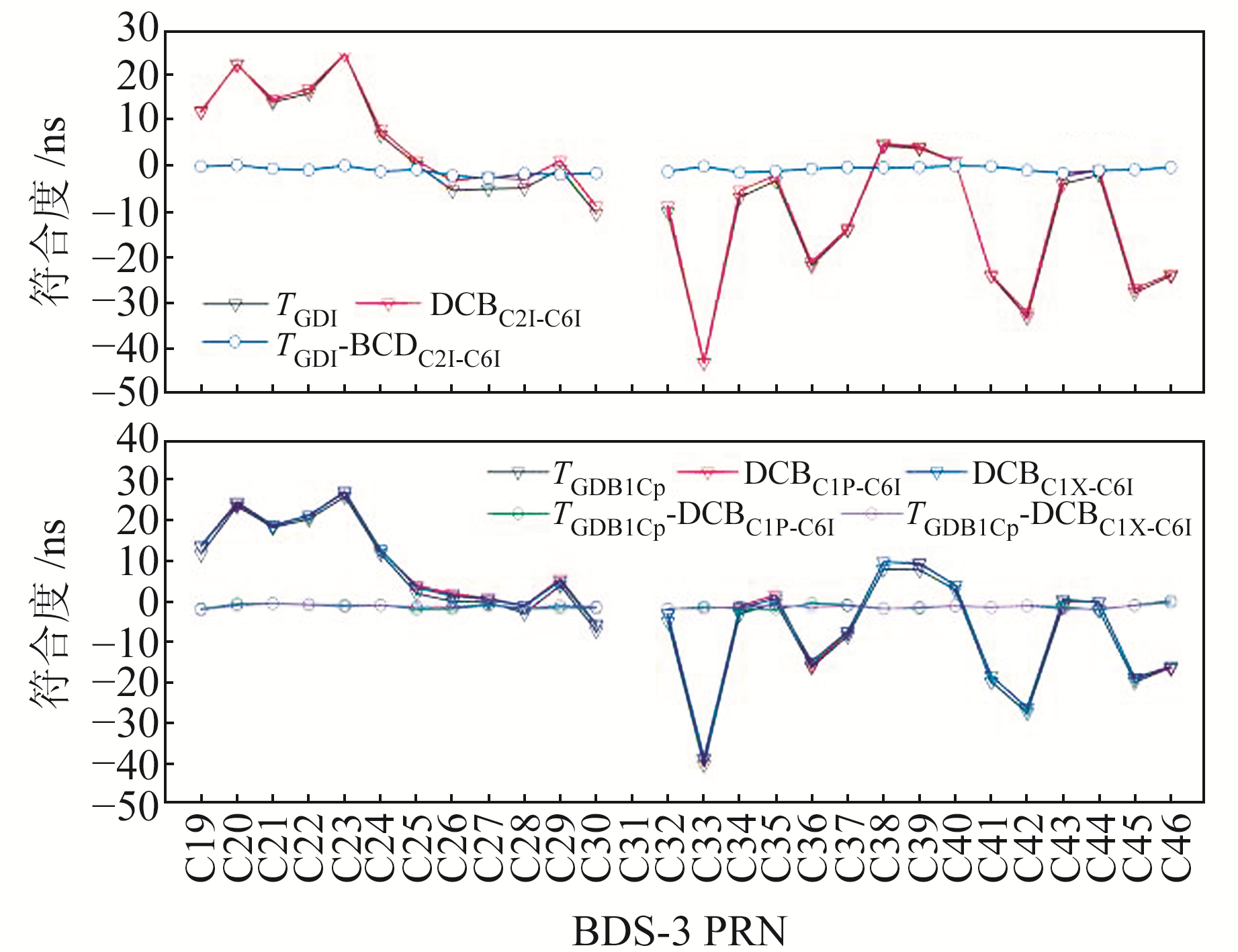图 2 BDS-3卫星TGD与DCB Fig. 2 BDS-3 satellite TGD and DCB values

2.3 可见卫星数及PDOP值表 1 平均可见卫星数和PDOP Tab. 1 Average number of visible stars and PDOP

2.4 单频SPP精度分析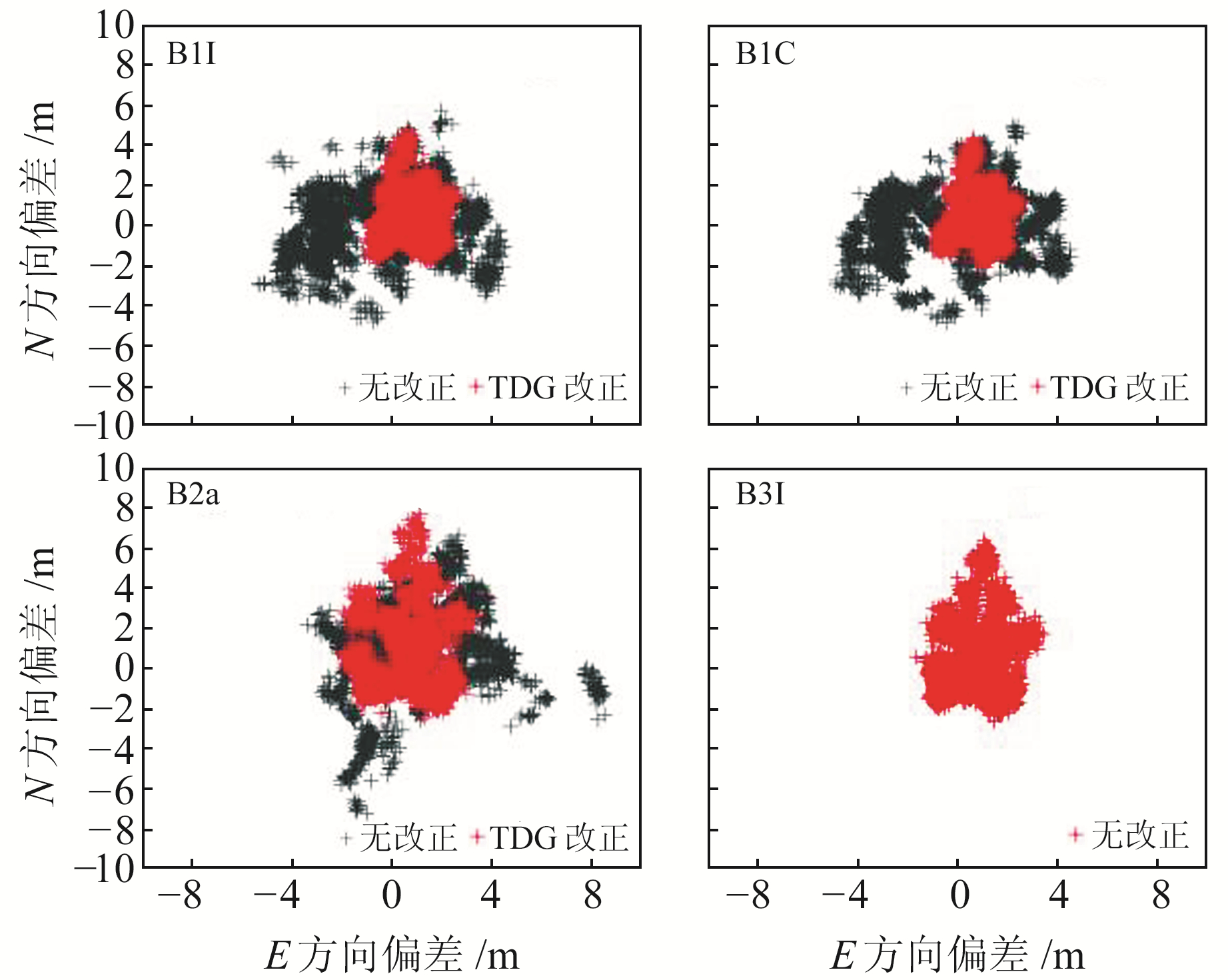图 3 NYA2站单频SPP水平方向定位偏差 Fig. 3 NYA2 station single-frequency SPP horizontalcomponent deviation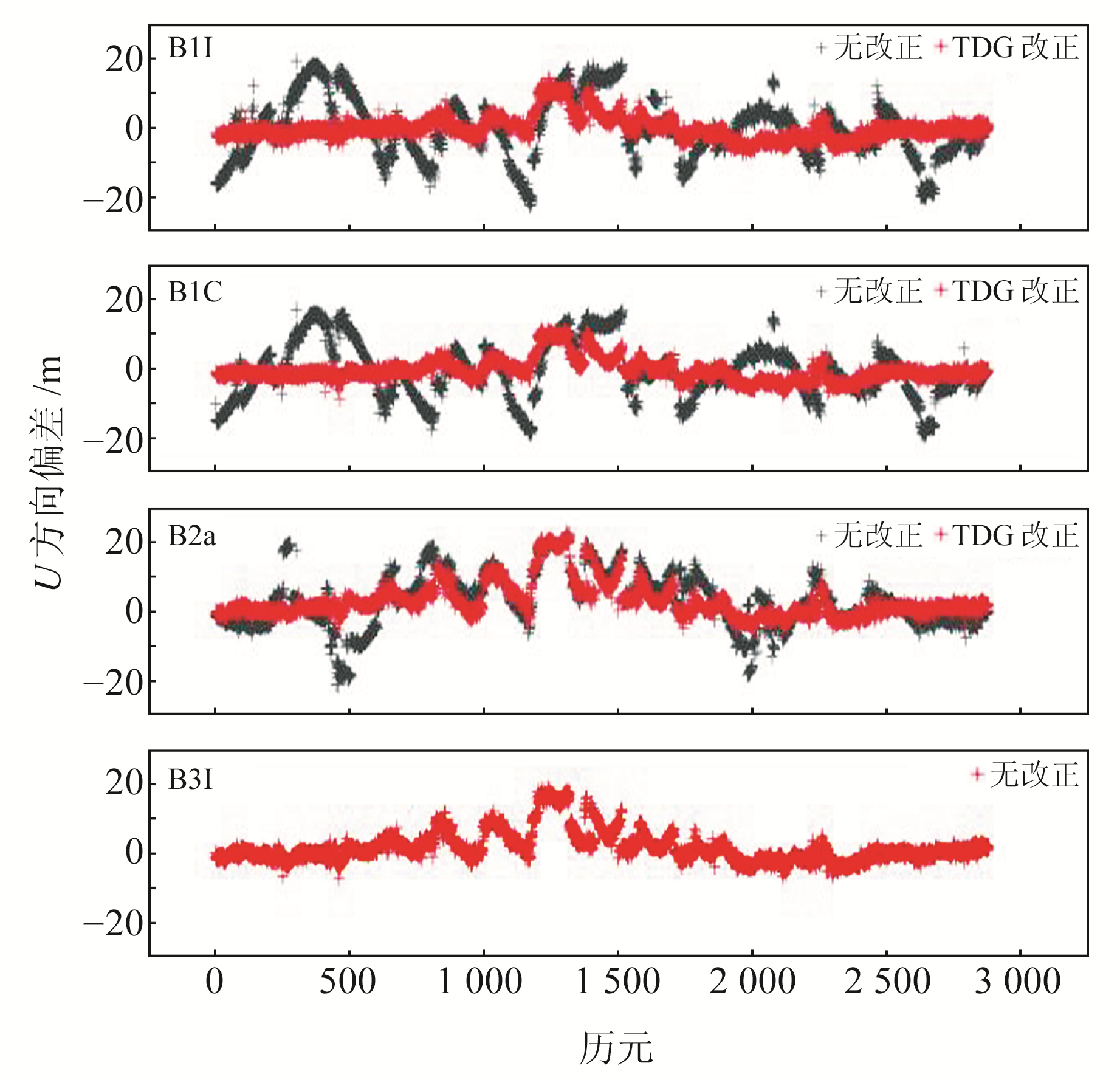图 4 NYA2站单频SPP高程方向定位偏差 Fig. 4 NYA2 station single-frequency SPP verticalcomponent positioning deviation表 2 单频SPP TGD改正前后定位精度 Tab. 2 Positioning accuracy before and after TGDcorrection of single-frequency SPP

2.5 双频SPP精度分析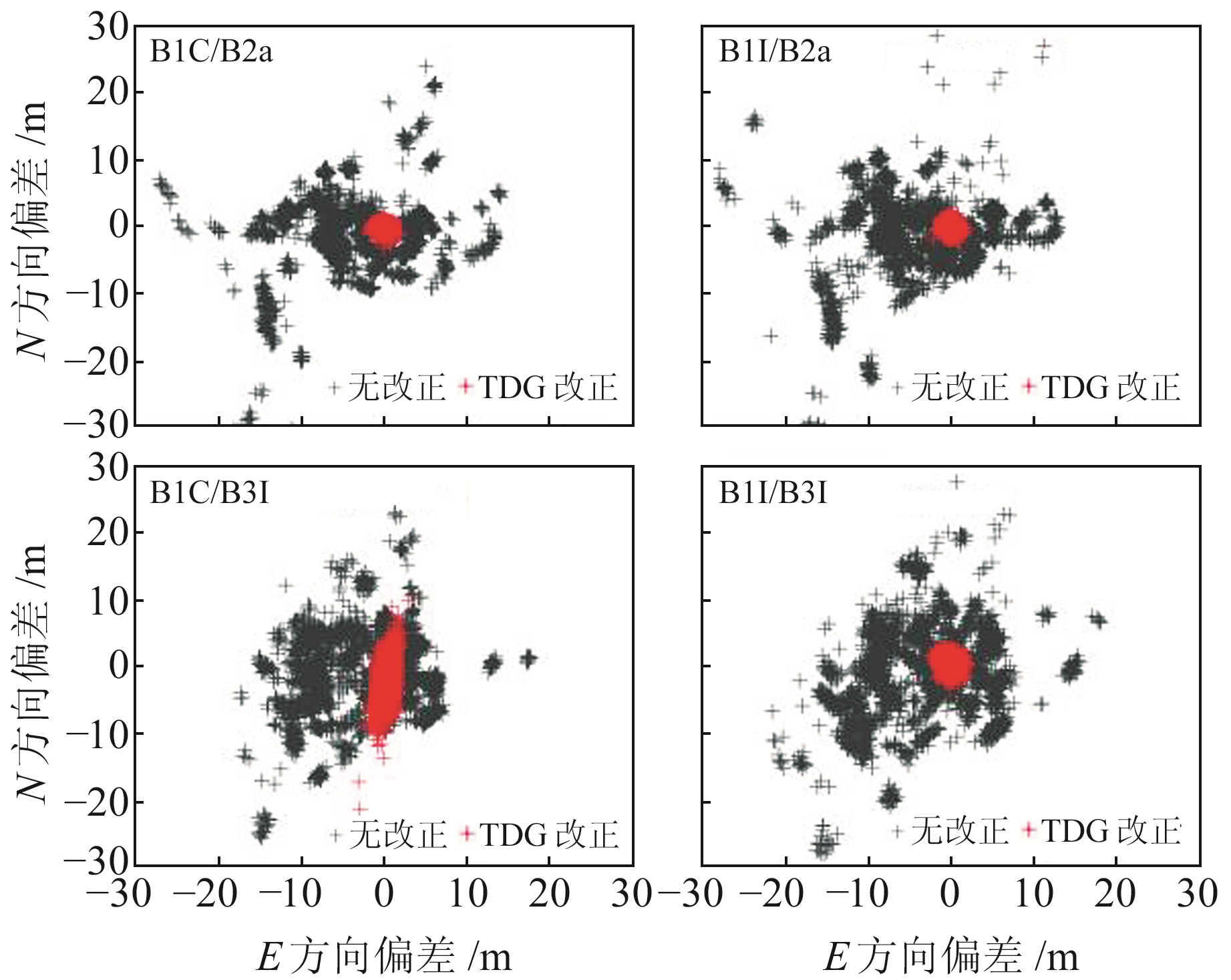图 5 NYA2站双频SPP水平方向定位偏差 Fig. 5 NYA2 station dual-frequency SPP horizontalcomponent deviation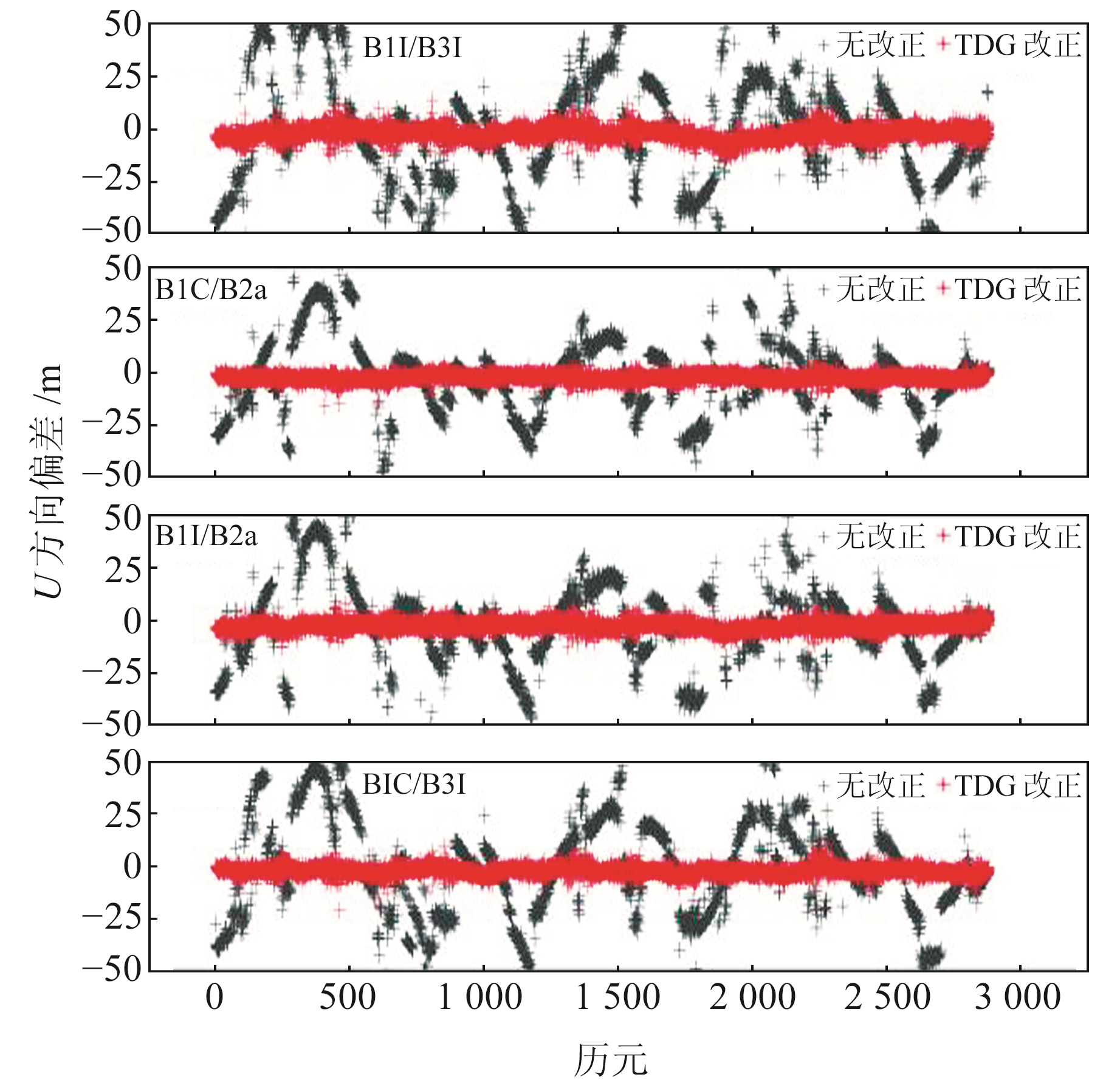图 6 NYA2站双频SPP高程方向定位偏差 Fig. 6 NYA2 station dual-frequency SPP verticalcomponent positioning deviation表 3 双频SPP TGD改正前后定位精度 Tab. 3 Positioning accuracy before and after TGD correction of dual-frequency SPP

3 结语

1) BDS-3的DCB产品在1个月内的日解值稳定，未出现明显跳变，稳定性为0.08~0.30 ns，大部分卫星的月稳定性优于0.2 ns，TGD参数与DCB产品符合度高，大部分卫星之间的差异优于2 ns。BDS-3平均可见卫星数基本为8~11颗、PDOP平均约为2，说明BDS-3具有稳定的可见卫星数，且空间几何构型良好。

2) 单频SPP经TGD改正后水平和高程方向的定位精度均有大幅提升，且水平方向的改正率优于高程方向。水平方向的定位精度由2~4 m提升至1~2 m，改正率为30%~70%；高程方向的定位精度由约8 m提升至3~5 m，提升率为17%~50%，其中B1I和B1C频点的改正效果优于B2a。B3I频点未经TGD改正，但其与其余频点改正后的定位精度基本相当，ENU方向的RMSE分别为1.17 m、1.46 m和4.67 m。

3) 与单频SPP相同，各组合双频SPP经TGD改正后水平及高程方向的定位精度均有大幅提升，且改正后组合间的定位精度相当，水平方向的定位精度由6~9 m提升至1 m左右，提升率约为90%；高程方向的定位精度由11 m左右改正至2.5~4 m，提升率为66%~71%。经TGD改正后各组合双频SPP的定位精度优于任意单频SPP，水平方向基本优于1 m，高程方向优于4 m。TGD改正对单、双频SPP定位精度的影响较大，在定位解算中不可忽略。

  王宁波, 袁运斌, 张宝成, 等. GPS民用广播星历中ISC参数精度分析及其对导航定位的影响[J]. 测绘学报, 2016, 45(8): 919-928 (Wang Ningbo, Yuan Yunbin, Zhang Baocheng, et al. Accuracy Evaluation of GPS Broadcast Inter-Signal Correction(ISC) Parameters and Their Impacts on GPS Standard Positioning[J]. Acta Geodaetica et Cartographica Sinica, 2016, 45(8): 919-928) (0)  Dai P P, Ge Y L, Qin W J, et al. BDS-3 Time Group Delay and Its Effect on Standard Point Positioning[J]. Remote Sensing, 2019, 11(15): 1 819 DOI:10.3390/rs11151819 (0)  Dai P P, Xing J P, Ge Y L, et al. The Effect of BDS-3 Time Group Delay and Differential Code Bias Corrections on Positioning[J]. Applied Sciences, 2020, 11(1): 104 DOI:10.3390/app11010104 (0)  Guo F, Zhang X H, Wang J L. Timing Group Delay and Differential Code Bias Corrections for Beidou Positioning[J]. Journal of Geodesy, 2015, 89(5): 427-445 DOI:10.1007/s00190-015-0788-2 (0)  Zhang Y Z, Wang H, Chen J P, et al. Calibration and Impact of Beidou Satellite-Dependent Timing Group Delay Bias[J]. Remote Sensing, 2020, 12(1): 192 DOI:10.3390/rs12010192 (0)  李坤, 王潜心, 龚佑兴, 等. 基于TGD/DCB改正的BDS多频单点定位精度分析[J]. 合肥工业大学学报: 自然科学版, 2021, 44(5): 685-690 (Li Kun, Wang Qianxin, Gong Youxing, et al. Accuracy Analysis on BDS Multi-Frequency Point Positioning Based on TGD/DCB Corrections[J]. Journal of Hefei University of Technology: Natural Science, 2021, 44(5): 685-690 DOI:10.3969/j.issn.1003-5060.2021.05.019) (0)  谷世铭, 党亚民, 王虎, 等. 北斗差分码偏差改正对单点定位的影响[J]. 测绘科学, 2020, 45(10): 10-15 (Gu Shiming, Dang Yamin, Wang Hu, et al. The Effect of Beidou DCB Correction on Single Point Positioning[J]. Science of Surveying and Mapping, 2020, 45(10): 10-15) (0)  刘生锋, 杨文龙, 谷涛. BDS-3双频组合精密单点定位精度分析[J]. 测绘通报, 2021(3): 7-11 (Liu Shengfeng, Yang Wenlong, Gu Tao. Accuracy Analysis of BDS-3 Dual Frequency Combination Precision Point Positioning[J]. Bulletin of Surveying and Mapping, 2021(3): 7-11) (0)  曾添, 隋立芬, 鲍亚东, 等. BDS卫星端差分码偏差对定位的影响及改正模型研究[J]. 大地测量与地球动力学, 2017, 37(1): 53-57 (Zeng Tian, Sui Lifen, Bao Yadong, et al. The Impact of Satellite Differential Code Bias on BDS Positioning and Correction Model Research[J]. Journal of Geodesy and Geodynamics, 2017, 37(1): 53-57) (0)  王利军, 焦文海, 贾小林, 等. BDS-3精密单点定位性能比较分析[J]. 大地测量与地球动力学, 2021, 41(4): 357-361 (Wang Lijun, Jiao Wenhai, Jia Xiaolin, et al. Comparative Analysis of BDS-3 Precise Point Positioning Performance[J]. Journal of Geodesy and Geodynamics, 2021, 41(4): 357-361) (0)  袁海军, 章浙涛, 何秀凤, 等. 北斗三号卫星差分码偏差稳定性分析及其对单点定位的影响[J]. 武汉大学学报: 信息科学版, 2023, 48(3): 425-432 (Yuan Haijun, Zhang Zhetao, He Xiufeng, et al. Stability Analysis of BDS-3 Satellite Differential Code Bias and Its Impacts on Single Point Positioning[J]. Geomatics and Information Science of Wuhan University, 2023, 48(3): 425-432) (0)
Influence of Timing Group Delay on BDS-3 New Signals Single Point Positioning
DU Yanjun1JIA Xiaolin2YAO Wanqiang1     LI Jiahao3
1. College of Geomatics, Xi'an University of Science and Technology, 58 Mid-Yanta Road, Xi'an 710054, China;
2. Xi'an Institute of Surveying and Mapping, 1 Mid-Yanta Road, Xi'an 710054, China;
3. School of Geological Engineering and Geomatics, Chang'an University, 126 Yanta Road, Xi'an 710054, China
Abstract: To explore the influence of BDS-3 single- and dual-frequency SPP TGD correction on positioning, we select the observation data of 10 MGEX stations for 30 days to carry out SPP experiments. The results show that the BDS-3 DCB product is stable within one month without noticeable jumps, and the monthly stability is better than 0.2 ns. The TGD parameters are more consistent with the DCB products, and most satellite differences are better than 2 ns. After TGD correction, the accuracy of single-frequency and dual-frequency SPP is greatly improved in both horizontal and vertical components. The improvement rate of single-frequency SPP is 17% to 70%, and dual-frequency SPP is 66% to 90%. The hardware delay deviation has a great influence on single point positioning and cannot be ignored in the positioning solution.
Key words: BDS-3; timing group delay; differential code bias; single point positioning; positioning accuracy